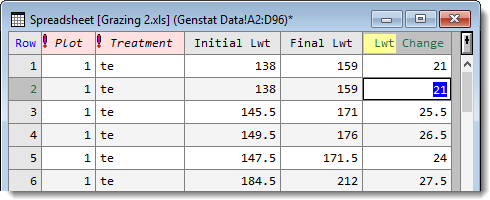1. Home
2. Calculating a Column

# Calculating a Column

Within Genstat calculations are performed on whole columns rather than cell by cell. As with Excel, you need to specify a formula or expression to perform the calculation. The Calculate dialog lets you create numerical or logical expressions to evaluate data in your spreadsheet and store the calculated results in a new column.

The empty field at the top of the calculator lets you construct your expression by combining data structures and operators. You can use brackets to control the order of evaluation when required.2. Select which columns you want to load into the Available data list by selecting the checkboxes for Variates, Factors, etc.
3. To construct your expression, double-click column names to copy them in. Type the operators directly into the expression or select them from the keypad.
4. Type a name for the resulting column in the Save result in field.

The images below show the result of calculating the difference between the two columns Initial Lwt and Final Lwt. The resulting column is called Lwt Change and is coloured half yellow to indicate that this is a calculated column.If you edit any of the values in the columns used to form the expression then update the server (for example, by clicking outside the spreadsheet), you will be prompted to recalculate the calculated column. You can turn off this prompt if desired by selecting Tools | Spreadsheet Options and clicking the General tab. Deselect the option Prompt to update calculated columns to disable this feature.Editing an expression

You can re-display and also edit the expression by double-clicking the calculated column’s header. This opens the Column Attributes/Format dialog.After editing an expression and clicking OK the following prompt will appear, allowing you to recalculate the column using the revised expression.Updated on June 20, 2019